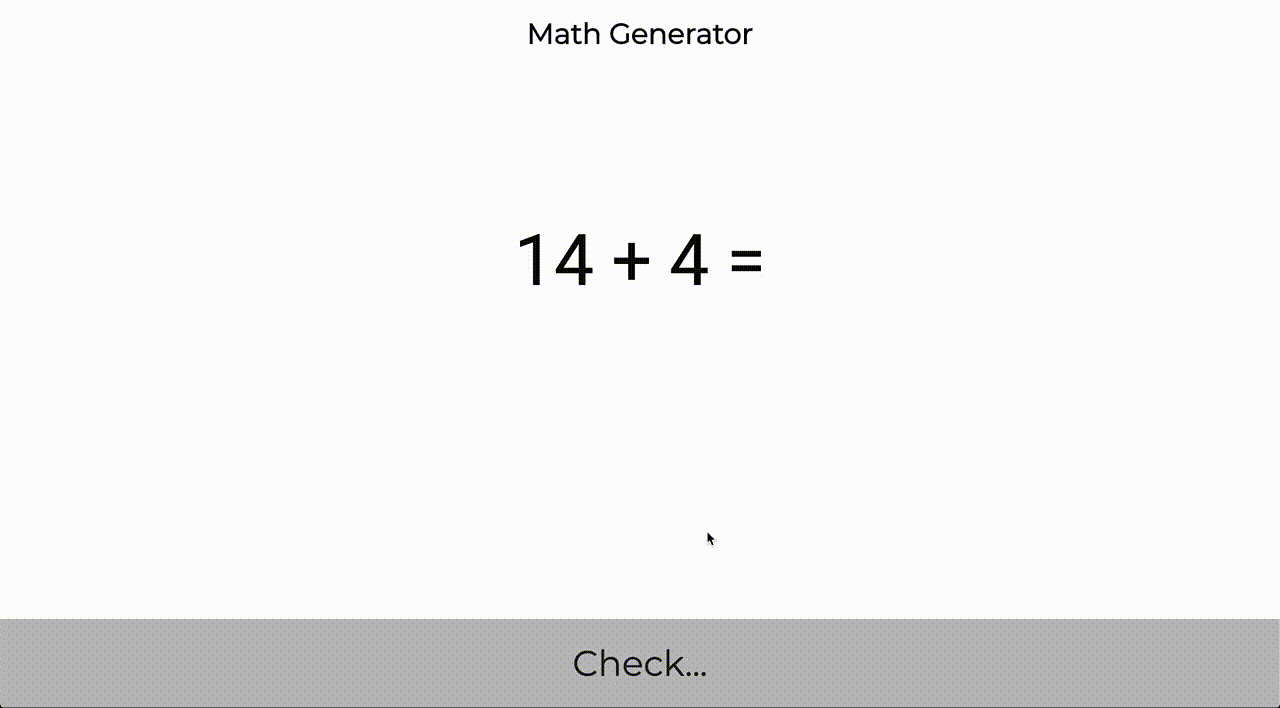Version: v0.1.0 Latest LatestGo to latest
Published: Jan 26, 2020 License: MITREADME ¶

Math generatorAs a dad, I frequently to play math quiz with my daughter. Bu I'm too lazy to frequently to create math questions. This' reason why I create this lib/api/web, that I can help me to generate questions. This repository will evolue frequently, I think, to support more topic.

Use as library

Install library:

go get -u github.com/ledongthuc/mathgen

Usage:

More detail supported functions is cover at: https://godoc.org/github.com/ledongthuc/mathgen

Run demo application

Startup

docker run -p 8080:8080 ledongthuc/mathgen-web:latest

Start from source code:

make clean; make test; make run;
API

Request:

{
"max_sum": 10
}

Response:

{
3,
4
],
"sum": 7,
"question": "3 + 4 = ",
"result": "3 + 4 = 7"
}
Subtraction

POST http://localhost:8080/api/subtraction/generate

Request:

{
"max_minuend": 20,
"number_of_subtrahends": 1
}

Response:

{
"minuend": 9,
"subtrahends": [
5
],
"difference": 4,
"question": "9 - 5 = ",
"result": "9 - 5 = 4"
}
Web

Local: http://localhost:8080

Free site: https://mathgen.thuc.spaceDocumentation ¶

Overview ¶

Package mathgen is a library for math's questions & answers generation.

Constants ¶

This section is empty.

Variables ¶

This section is empty.

Functions ¶

This section is empty.

Types ¶

Sum     int64
}

AddIntegerN generate a operator with sum. Parameter numberOfAddends defines addends in question, it should be greater than 1. Parameter maxSum defines maxsimum of Sum result, it should be greater than 0. If maxSum is greater than or equal numberOfAddends. Question always has pattern: 1 + 1 + 1 ....

Example
package main

import (
"fmt"

"github.com/ledongthuc/mathgen"
)

func main() {
}
Output:

AddIntegers generate a operator with sum of 2 addends. Parameter maxSum defines maxsimum of Sum result, it should be greater than 0. If maxSum = 2, addends always is 1 + 1

Example
package main

import (
"fmt"

"github.com/ledongthuc/mathgen"
)

func main() {
}
Output:

String of AdditionResult will present struct data with question answer format a + b + c + ... = sum

StringQuestion of AdditionResult will present struct data with question format a + b + c + ... =

type SubtractionResult ¶

type SubtractionResult struct {
Minuend     int64
Subtrahends []int64
Difference  int64
}

func SubtractIntegerN ¶

func SubtractIntegerN(numberOfSubtrahends int, maxMinuend int64) (SubtractionResult, error)

SubtractIntegerN generate a operator with subtraction. Parameter numberOfSubtrahends defines subtrahends in question, it should be greater than 0. Parameter maxMinuend defines maxsimum of minuend result, it should be greater than 0. If maxMinuend = 1, addends always is 1 - 1 - 0 ....

Example
package main

import (
"fmt"

"github.com/ledongthuc/mathgen"
)

func main() {
}
Output:

func SubtractIntegers ¶

func SubtractIntegers(maxMinuend int64) (SubtractionResult, error)

SubtractIntegers generate a operator with subtraction of 1 minuend and 1 subtrahend. Parameter maxMinuend defines maxsimum of minuend, it should be greater than 0. If maxMinuend = 1, addends always is 1 - 1

Example
package main

import (
"fmt"

"github.com/ledongthuc/mathgen"
)

func main() {
}
Output:

func (SubtractionResult) String ¶

func (s SubtractionResult) String() string

String of SubtractionResult will present struct data with question answer format a - b - c - d ... = difference

func (SubtractionResult) StringQuestion ¶

func (s SubtractionResult) StringQuestion() string

StringQuestion of SubtractionResult will present struct data with question format a - b - c - d ... =

Path Synopsis